# Mapping fingerprint data into 2D¶

Inspired by this notebook from George Papadatos.

Scikit-learn makes it quite easy to apply multi-dimensional scaling (MDS) to either reduce the dimensionality of a dataset or to embed distance data into cartesian space. This enables one of the favorite activities of the cheminformatician: producing plots of where compounds land in an 2D space. Things like this one, embedding a set of ~120 compounds with Serine-protein kinase ATR data in ChEMBL: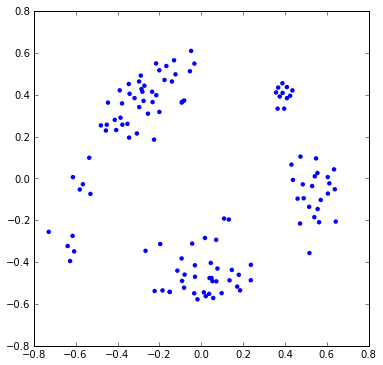Here I'm going to take a look at how accurate these embeddings are and how sensitive that accuracy is to the embedding method, dataset size, and dataset composition.

TL;DR Version

Here's a plot of the Euclidean distance in the projected 2D space vs the original Tanimoto distance for George's compounds: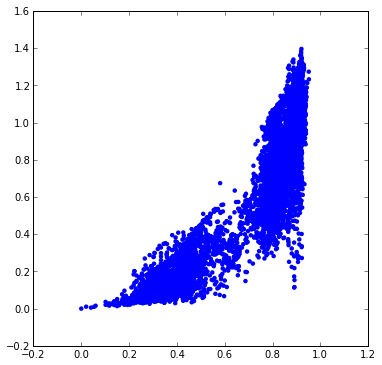There are clearly a lot of distances that are not being accurately represented. It is somewhat encouraging that no distances that should be small (<0.4) end up being large, but the correlation really isn't all that great.

By plotting residual strain per point versus dimension: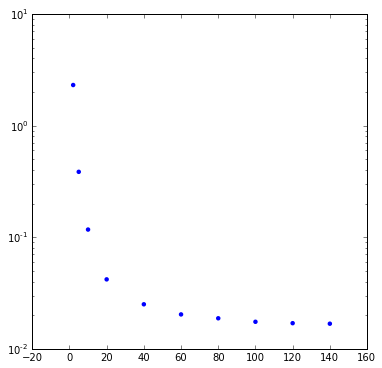it becomes clear that, at least according to the MDS, this dataset really isn't 2D.

# On to the analysis¶

In :
```import requests

import pandas as pd

from rdkit.Chem.Draw import IPythonConsole
from rdkit.Chem import PandasTools
from rdkit.Chem import AllChem as Chem
from rdkit.Chem import DataStructs

from scipy.spatial.distance import *
import numpy as np
from sklearn import manifold

from rdkit import rdBase
print(rdBase.rdkitVersion)

import time
print time.asctime()
```
```2014.03.1pre
Mon Jan 27 04:07:13 2014

```

## Grab the data

I'm going to start with a larger dataset so that I can explore the impact of dataset size on the results. The analysis of George's results are below.

I'll use the Dopamine D3 receptor as the target for this exercise. It's one of the targets we used in both the benchmarking and model fusion papers.

In :
```target='CHEMBL234'
re = requests.get('https://www.ebi.ac.uk/chemblws/targets/{0}/bioactivities.json'.format(target))
data = pd.DataFrame(re.json()['bioactivities'])
data.head()
```
Out:
activity_comment assay_chemblid assay_description assay_type bioactivity_type ingredient_cmpd_chemblid name_in_reference operator organism parent_cmpd_chemblid reference target_chemblid target_confidence target_name units value
0 Not Determined CHEMBL860513 Agonist activity at human D3 receptor expressed in CHO dhfr- cells assessed as rate of [3H]thymidine incorporation relative to quinpirole by mitogenesis assay F Activity CHEMBL208847 4 Unspecified Homo sapiens CHEMBL208847 J. Med. Chem., (2006) 49:12:3628 CHEMBL234 9 Dopamine D3 receptor Unspecified Unspecified
1 Unspecified CHEMBL897620 Displacement of [3H]spiperone from human D3 receptor expressed in CHO cells B Ki CHEMBL393774 16c > Homo sapiens CHEMBL393774 Bioorg. Med. Chem., (2007) 15:23:7258 CHEMBL234 9 Dopamine D3 receptor nM 1000
2 Not Determined CHEMBL860514 Intrinsic activity at human D3 receptor expressed in CHO dhfr- cells assessed as rate of [3H]thymidine incorporation by mitogenesis assay F EC50 CHEMBL208847 4 Unspecified Homo sapiens CHEMBL208847 J. Med. Chem., (2006) 49:12:3628 CHEMBL234 9 Dopamine D3 receptor Unspecified Unspecified
3 Unspecified CHEMBL669163 Binding affinity towards human cloned dopamine (hD3) receptor expressed in CHO cells using [3H]spiperone as radioligand B Ki CHEMBL101032 15 > Homo sapiens CHEMBL101032 Bioorg. Med. Chem. Lett., (1998) 8:3:295 CHEMBL234 8 Dopamine D3 receptor nM 400
4 Unspecified CHEMBL673116 Binding affinity at Dopamine receptor D3 expressed in CHO cells by [125I]iodosulpiride displacement. B Log Ki CHEMBL2111592 2c = Homo sapiens CHEMBL2111592 Bioorg. Med. Chem. Lett., (1998) 8:20:2859 CHEMBL234 8 Dopamine D3 receptor Unspecified 8
In :
```data.shape
```
Out:
```(6499, 16)
```

## Remove duplicates

In :
```data = data.drop_duplicates(['parent_cmpd_chemblid'])
data.shape
```
Out:
```(4269, 16)
```

## Remove assays with too many or too few compounds

In :
```assays = data[['assay_chemblid','target_chemblid']].groupby('assay_chemblid').count()
print assays.shape
assays.head(5)
```
```(460, 2)

```
Out:
assay_chemblid target_chemblid
assay_chemblid
CHEMBL1030601 10 10
CHEMBL1030747 1 1
CHEMBL1031083 5 5
CHEMBL1031401 1 1
CHEMBL1032032 1 1
In :
```assays.assay_chemblid.hist(bins=20)
```
Out:
```<matplotlib.axes.AxesSubplot at 0x6832c50>
```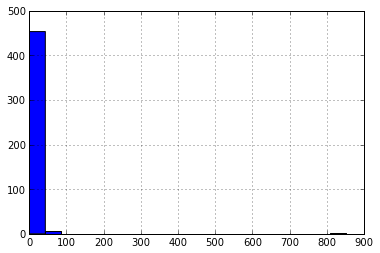In :
```goodassays = assays.ix[(assays.assay_chemblid >= 10)&(assays.assay_chemblid <= 30)]
print goodassays.shape
goodassays.assay_chemblid.hist(bins=20)
```
```(104, 2)

```
Out:
```<matplotlib.axes.AxesSubplot at 0x67ef290>
```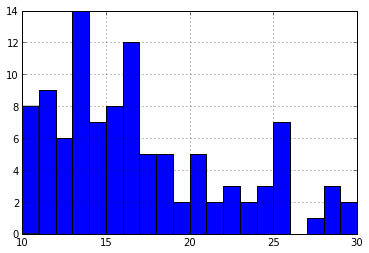In :
```data2 = data.ix[data.assay_chemblid.isin(list(goodassays.index))]
data2.shape
```
Out:
```(1728, 16)
```

## Now get the SMILES

Note: George's original notebook used the ChEMBL web services to grab the structures. Since the ChEMBL web services don't seem to have any way to do bulk retrieval, this requires one call per compound, which takes a long time. For larger datasets it's almost definitely a better idea to do queries against a local copy of ChEMBL, so that's what we'll do here.

In :
```%load_ext sql
%config SqlMagic.feedback = False
```
In :
```def fetch_SMILES(chemblid):
data = %sql postgresql://localhost/chembl_16 \
select canonical_smiles smiles from compound_structures \
join molecule_dictionary using (molregno) \
where chembl_id=:chemblid ;
return data
```
In :
```data2['SMILES'] = data2['parent_cmpd_chemblid'].map(fetch_SMILES)
```
In :
```PandasTools.AddMoleculeColumnToFrame(data2, smilesCol = 'SMILES',includeFingerprints=True)
```

Let's start by looking at assays that have between 10 and 12 compounds:

In :
```assays = data2[['assay_chemblid','target_chemblid']].groupby('assay_chemblid').count()
print assays.shape
goodassays = assays.ix[(assays.assay_chemblid >= 10)&(assays.assay_chemblid <= 12)]
subset = data2.ix[data.assay_chemblid.isin(list(goodassays.index))]
print subset.shape
```
```(104, 2)
(251, 18)

```
In :
```mols = subset[['parent_cmpd_chemblid','name_in_reference','SMILES', 'ROMol', 'assay_chemblid']]
mols.shape
```
Out:
```(251, 5)
```
In :
```mols.head(5)
```
Out:
parent_cmpd_chemblid name_in_reference SMILES ROMol assay_chemblid
15 CHEMBL269202 10e Clc1cccc(c1)N2CCN(Cc3cnn4ccccc34)CC2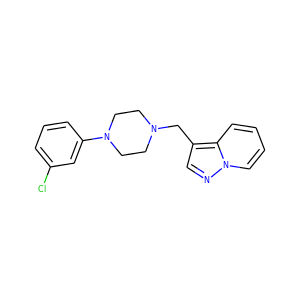CHEMBL673448
188 CHEMBL2219587 22 O=C(N[C@@H]1CC[C@@H](CCN2CCc3cc(ccc3C2)C#N)CC1)c4ccc5ccccc5c4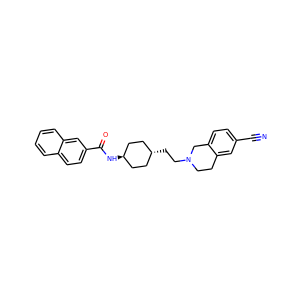CHEMBL679078
198 CHEMBL1774544 13b CCCN(CCCCN1CCN(CC1)c2cccc(Cl)c2Cl)Cc3csc4ccccc34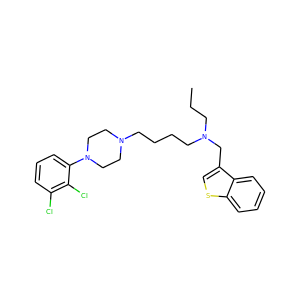CHEMBL1777179
243 CHEMBL250552 6a CCCN(CCN1CCN(CC1)c2ccccc2)C3CCc4c(O)cccc4C3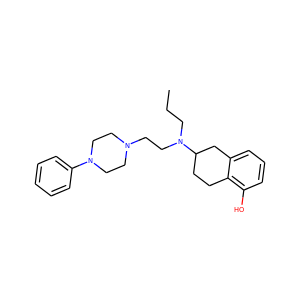CHEMBL926380
248 CHEMBL2165679 17 CCC(=O)NCCCCN1CCN(CC1)c2ccccc2OC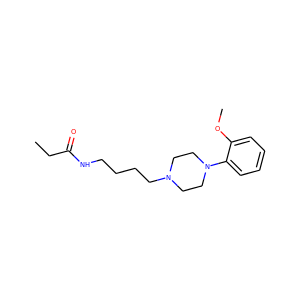CHEMBL2167999

Construct fingerprints:

In :
```fps = [Chem.GetMorganFingerprintAsBitVect(m,2,nBits=2048) for m in mols['ROMol']]
```

Now the distance matrix:

In :
```dist_mat = []
for i,fp in enumerate(fps):
dist_mat.append(DataStructs.BulkTanimotoSimilarity(fps[i],fps,returnDistance=1))
dist_mat=numpy.array(dist_mat)

```

And now do the MDS into 2 dimensions:

In :
```mds = manifold.MDS(n_components=2, dissimilarity="precomputed", random_state=3, n_jobs = 4, verbose=1,max_iter=1000)
results = mds.fit(dist_mat)
coords = results.embedding_
print 'Final stress:',mds.stress_
```
```Final stress: 2981.10141187
breaking at iteration 213 with stress 2990.11126018
breaking at iteration 242 with stress 2981.10141187
breaking at iteration 266 with stress 3001.72037202
breaking at iteration 291 with stress 3035.55798538

```

And plot the points:

In :
```rcParams['figure.figsize'] = 6,6
```
In :
```scatter([x for x,y in coords], [y for x,y in coords],edgecolors='none')
```
Out:
```<matplotlib.collections.PathCollection at 0xa2b8f50>
```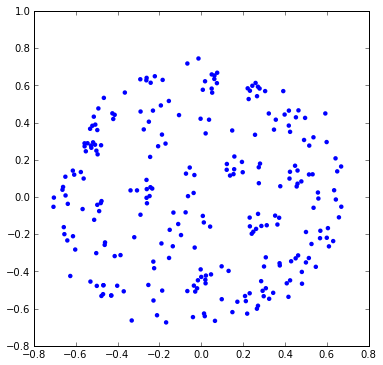Nice picture. How accurate is it?

The strain values above lead me to believe that there are probably some real problems here.

The overall strain value isn't particularly easy to interpret, so we'll check up on things by comparing the embedded distances with the Tanimoto distances we're trying to reproduce.

In :
```import random
def distCompare(dmat,coords,nPicks=5000,seed=0xf00d):
""" picks a random set of pairs of points to compare distances """
nPts=len(coords)
random.seed(seed)
res=[]
keep=set()
if nPicks>0:
while len(res)<nPicks:
idx1 = random.randint(0,nPts-1)
idx2 = random.randint(0,nPts-1)
if idx1==idx2:
continue
if idx1>idx2:
idx1,idx2=idx2,idx1
if (idx1,idx2) in keep:
continue
keep.add((idx1,idx2))
p1 = coords[idx1]
p2 = coords[idx2]
v = p1-p2
d = sqrt(v.dot(v))
res.append((dmat[idx1][idx2],d))
else:
for idx1 in range(nPts):
for idx2 in range(idx1+1,nPts):
p1 = coords[idx1]
p2 = coords[idx2]
v = p1-p2
d = sqrt(v.dot(v))
res.append((dmat[idx1][idx2],d))
return res
```
In :
```d = distCompare(dist_mat,coords)
```
In :
```scatter([x for x,y in d],[y for x,y in d],edgecolors='none')
```
Out:
```<matplotlib.collections.PathCollection at 0xa6b6590>
```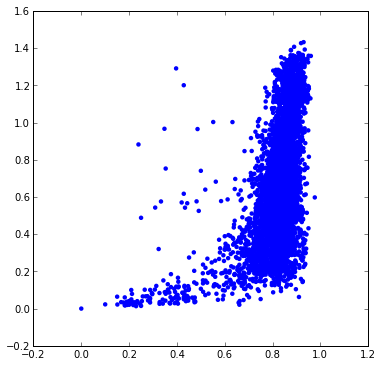Yikes... that's no good. This really isn't so surprising given how much stress was left.

Let's try increasing the dimensionality to see how much improvement we can get:

In :
```mds2 = manifold.MDS(n_components=20, dissimilarity="precomputed", random_state=3, n_jobs = 4, verbose=1,max_iter=1000)
results = mds2.fit(dist_mat)
coords = results.embedding_
print 'Final stress:',mds2.stress_
```
```Final stress: 60.0722043196
breaking at iteration 204 with stress 62.4491504306
breaking at iteration 207 with stress 62.2071008137
breaking at iteration 209 with stress 63.1637381106
breaking at iteration 213 with stress 60.0722043196

```
In :
```d = distCompare(dist_mat,coords)
scatter([x for x,y in d],[y for x,y in d],edgecolors='none')
```
Out:
```<matplotlib.collections.PathCollection at 0xa9dddd0>
```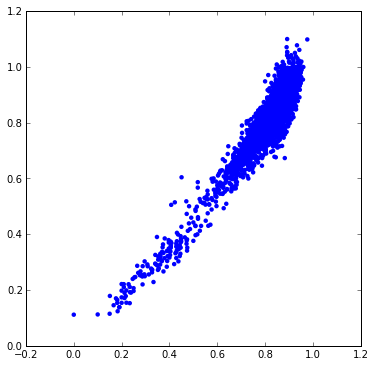Not so bad... of course, we can't visualize 20 dimensions.

What about if we try doing dimensionality reduction on the 20D results instead of using the distance matrix?

In :
```mds3 = manifold.MDS(n_components=2, random_state=3, n_jobs = 4, verbose=1,max_iter=1000)
results3 = mds3.fit(coords)
coords3 = results3.embedding_
print 'Final stress:',mds3.stress_
```
```Final stress: 2820.95425215
breaking at iteration 227 with stress 2908.54991275
breaking at iteration 307 with stress 2904.20719109
breaking at iteration 358 with stress 2987.18251943
breaking at iteration 360 with stress 2820.95425215

```

The strain is still really high. This isn't that surprising given that we already tried the same thing from the distance matrix.

Ok, so MDS doesn't work. Now that we have actual coordinates, we can use some of the other scikit-learn manifold learning approaches for embedding the points.

Instead of jumping all the way to a 2D system, where we're really unlikely to get decent results, start by dropping down to 10D.

First MDS in 10D:

In :
```mds3 = manifold.MDS(n_components=10, random_state=3, n_jobs = 4, verbose=1,max_iter=1000)
results3 = mds3.fit(coords)
coords3 = results3.embedding_
print 'Final stress:',results3.stress_
ds = distCompare(dist_mat,coords3)
scatter([x for x,y in d],[y for x,y in d],edgecolors='none')
```
```Final stress: 164.796744564
breaking at iteration 200 with stress 169.113855631
breaking at iteration 205 with stress 164.796744564
breaking at iteration 213 with stress 168.607644481
breaking at iteration 214 with stress 173.12479574

```
Out:
```<matplotlib.collections.PathCollection at 0xaac9410>
```Now try locally linear embedding:

In :
```lle = manifold.LocallyLinearEmbedding(n_components=10,random_state=3,max_iter=1000)
results3=lle.fit(coords)
coords3=results3.embedding_
print results3.reconstruction_error_
d = distCompare(dist_mat,coords3)
scatter([x for x,y in d],[y for x,y in d],edgecolors='none')
```
```9.51048182171e-16

```
Out:
```<matplotlib.collections.PathCollection at 0xaf3ce10>
```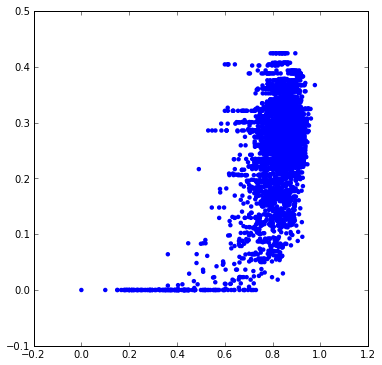Ick! What about Isomap?

In :
```embed = manifold.Isomap(n_components=10,max_iter=1000)
results3=embed.fit(coords)
coords3=results3.embedding_
d = distCompare(dist_mat,coords3)
scatter([x for x,y in d],[y for x,y in d],edgecolors='none')
```
Out:
```<matplotlib.collections.PathCollection at 0xb244ad0>
```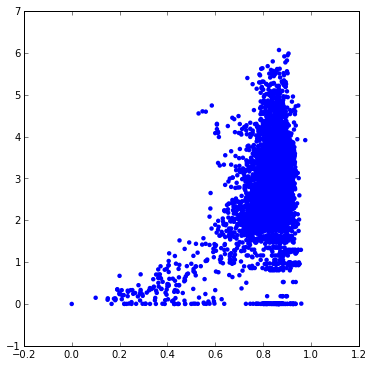Also pretty grim. Does spectral embedding work?

In :
```embed = manifold.SpectralEmbedding(n_components=10)
results3=embed.fit(coords)
coords3=results3.embedding_
d = distCompare(dist_mat,coords3)
scatter([x for x,y in d],[y for x,y in d],edgecolors='none')
```
Out:
```<matplotlib.collections.PathCollection at 0xb02cfd0>
```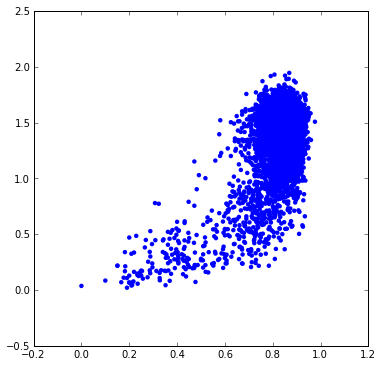Hmm, there's at least some signal there. How does spectral embedding do with 2D?

In :
```embed = manifold.SpectralEmbedding(n_components=2)
results3=embed.fit(coords)
coords3=results3.embedding_
d = distCompare(dist_mat,coords3)
scatter([x for x,y in d],[y for x,y in d],edgecolors='none')
```
Out:
```<matplotlib.collections.PathCollection at 0xb0587d0>
```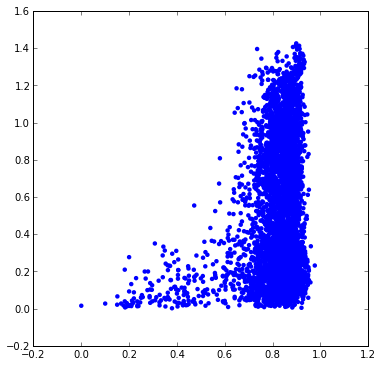Not so hot...

# Look at stress vs dimension

We're able to do a reasonable job of embedding into 20 dimensions with MDS. 10 even looks somewhat ok. Let's look at behavior of the residual stress as a function of dimension:

In :
```vs={}
for nComps in (140,120,100,80,60,40,20,10,5,2):
mds3 = manifold.MDS(n_components=nComps, random_state=3, dissimilarity="precomputed",n_jobs = 4, verbose=0,max_iter=1000)
results3 = mds3.fit(dist_mat)
print 'Final stress at dim=%d:'%nComps,results3.stress_,'stress per element:',results3.stress_/len(dist_mat)
vs[nComps]=results3.stress_/len(dist_mat)
scatter(vs.keys(),vs.values(),edgecolors='none')
yscale('log')
```
```Final stress at dim=140: 11.9512660916 stress per element: 0.0416420421311
Final stress at dim=120: 12.5917716347 stress per element: 0.043873768762

```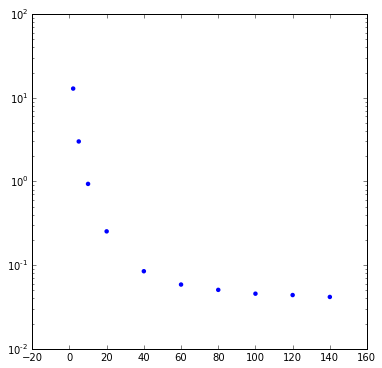Yeah, the stress is going up exponentially with dimension. These data really don't want to be in a 2D space.

Try non-metric embedding

In :
```vs={}
for nComps in (140,120,100,80,60,40,20,10,5,2):
mds3 = manifold.MDS(metric=False,n_components=nComps,random_state=3, dissimilarity="precomputed",n_jobs = 4, verbose=0,max_iter=1000)
results3 = mds3.fit(dist_mat)
print 'Final stress at dim=%d:'%nComps,results3.stress_,'stress per element:',results3.stress_/len(dist_mat)
vs[nComps]=results3.stress_/len(dist_mat)
scatter(vs.keys(),vs.values(),edgecolors='none')
yscale('log')
```
```Final stress at dim=140: 18.4515773264 stress per element: 0.0642912101966
Final stress at dim=120: 21.5234534437 stress per element: 0.074994611302

```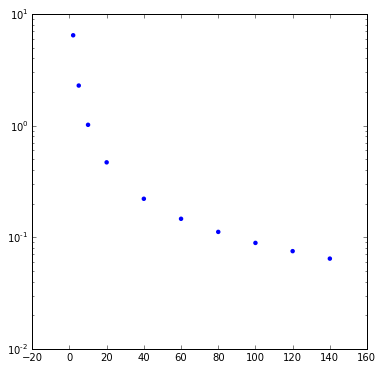helps somewhat at lower dimension, but not dramatically. Plus it's a lot slower

# A larger data set

Maybe it's possible to do better with a larger data set? This seems unlikely, but we can at least try:

In :
```assays = data2[['assay_chemblid','target_chemblid']].groupby('assay_chemblid').count()
print assays.shape
goodassays = assays.ix[(assays.assay_chemblid >= 10)&(assays.assay_chemblid <= 15)]
subset = data2.ix[data.assay_chemblid.isin(list(goodassays.index))]
print subset.shape
```
```(103, 2)
(660, 18)

```
In :
```mols = subset[['parent_cmpd_chemblid','name_in_reference','SMILES', 'ROMol', 'assay_chemblid']]
print mols.shape
fps = [Chem.GetMorganFingerprintAsBitVect(m,2,nBits=2048) for m in mols['ROMol']]
dist_mat = []
for i,fp in enumerate(fps):
dist_mat.append(DataStructs.BulkTanimotoSimilarity(fps[i],fps,returnDistance=1))
dist_mat=numpy.array(dist_mat)
print dist_mat.shape
```
```(660, 5)
(660, 660)

```
In :
```vs={}
for nComps in (140,120,100,80,60,40,20,10,5,2):
mds3 = manifold.MDS(n_components=nComps, random_state=3, dissimilarity="precomputed",n_jobs = 4, verbose=0,max_iter=1000)
results3 = mds3.fit(dist_mat)
print 'Final stress at dim=%d:'%nComps,results3.stress_,'stress per element:',results3.stress_/len(dist_mat)
vs[nComps]=results3.stress_/len(dist_mat)
scatter(vs.keys(),vs.values(),edgecolors='none')
yscale('log')
```
```Final stress at dim=140: 59.6641705869 stress per element: 0.090400258465
Final stress at dim=120: 64.0164562249 stress per element: 0.0969946306437

```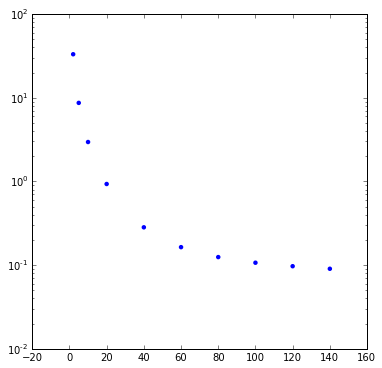Nope, that definitely made things worse.

# and larger still

In :
```assays = data2[['assay_chemblid','target_chemblid']].groupby('assay_chemblid').count()
print assays.shape
goodassays = assays.ix[(assays.assay_chemblid >= 10)&(assays.assay_chemblid <= 18)]
subset = data2.ix[data.assay_chemblid.isin(list(goodassays.index))]
print subset.shape
```
```(103, 2)
(962, 18)

```
In :
```mols = subset[['parent_cmpd_chemblid','name_in_reference','SMILES', 'ROMol', 'assay_chemblid']]
print mols.shape
fps = [Chem.GetMorganFingerprintAsBitVect(m,2,nBits=2048) for m in mols['ROMol']]
dist_mat = []
for i,fp in enumerate(fps):
dist_mat.append(DataStructs.BulkTanimotoSimilarity(fps[i],fps,returnDistance=1))
dist_mat=numpy.array(dist_mat)
print dist_mat.shape
```
```(962, 5)
(962, 962)

```
In :
```vs={}
for nComps in (140,120,100,80,60,40,20,10,5,2):
mds3 = manifold.MDS(n_components=nComps, random_state=3, dissimilarity="precomputed",n_jobs = 4, verbose=0,max_iter=1000)
results3 = mds3.fit(dist_mat)
print 'Final stress at dim=%d:'%nComps,results3.stress_,'stress per element:',results3.stress_/len(dist_mat)
vs[nComps]=results3.stress_/len(dist_mat)
scatter(vs.keys(),vs.values(),edgecolors='none')
yscale('log')
```
```Final stress at dim=140: 122.234861114 stress per element: 0.127063265192
Final stress at dim=120: 131.431566958 stress per element: 0.136623250476

```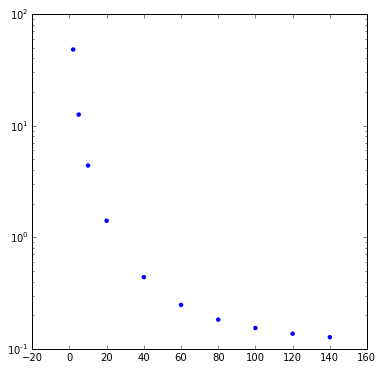Continuing to get worse.

# Try less data

In :
```assays = data2[['assay_chemblid','target_chemblid']].groupby('assay_chemblid').count()
print assays.shape
goodassays = assays.ix[assays.assay_chemblid == 15]
subset = data2.ix[data.assay_chemblid.isin(list(goodassays.index))]
print subset.shape
```
```(103, 2)
(105, 18)

```
In :
```mols = subset[['parent_cmpd_chemblid','name_in_reference','SMILES', 'ROMol', 'assay_chemblid']]
print mols.shape
fps = [Chem.GetMorganFingerprintAsBitVect(m,2,nBits=2048) for m in mols['ROMol']]
dist_mat = []
for i,fp in enumerate(fps):
dist_mat.append(DataStructs.BulkTanimotoSimilarity(fps[i],fps,returnDistance=1))
dist_mat=numpy.array(dist_mat)
print dist_mat.shape
```
```(105, 5)
(105, 105)

```
In :
```vs={}
for nComps in (140,120,100,80,60,40,20,10,5,2):
mds3 = manifold.MDS(n_components=nComps, random_state=3, dissimilarity="precomputed",n_jobs = 4, verbose=0,max_iter=1000)
results3 = mds3.fit(dist_mat)
print 'Final stress at dim=%d:'%nComps,results3.stress_,'stress per element:',results3.stress_/len(dist_mat)
vs[nComps]=results3.stress_/len(dist_mat)
scatter(vs.keys(),vs.values(),edgecolors='none')
yscale('log')
```
```Final stress at dim=140: 1.99539499633 stress per element: 0.0190037618698
Final stress at dim=120: 2.02795956996 stress per element: 0.0193139006663

```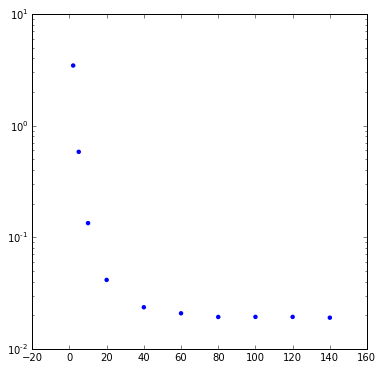That's a lot less stress per point than before, but it's still pretty high. Look at the distances to be sure:

In :
```mds3 = manifold.MDS(n_components=2, random_state=3, dissimilarity="precomputed",n_jobs = 4, verbose=0,max_iter=1000)
results3 = mds3.fit(dist_mat)
coords3=results3.embedding_
d = distCompare(dist_mat,coords3)
scatter([x for x,y in d],[y for x,y in d],edgecolors='none')
```
Out:
```<matplotlib.collections.PathCollection at 0xb9b4e50>
```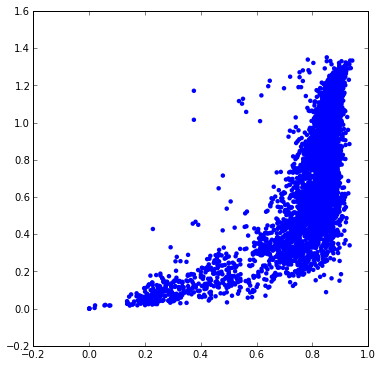# Embedding 100 random compounds:

In :
```data = %sql postgresql://localhost/chembl_16 \
select canonical_smiles smiles from compound_structures limit 50000 ;
random.seed(0xf00d)
random.shuffle(data)
smis = data[:100]
```
In :
```mols = [Chem.MolFromSmiles(x) for x in smis]
fps = [Chem.GetMorganFingerprintAsBitVect(m,2,nBits=2048) for m in mols]
dist_mat = []
for i,fp in enumerate(fps):
dist_mat.append(DataStructs.BulkTanimotoSimilarity(fps[i],fps,returnDistance=1))
dist_mat=numpy.array(dist_mat)
print dist_mat.shape
```
```(100, 100)

```
In :
```vs={}
for nComps in (140,120,100,80,60,40,20,10,5,2):
mds3 = manifold.MDS(n_components=nComps, random_state=3, dissimilarity="precomputed",n_jobs = 4, verbose=0,max_iter=1000)
results3 = mds3.fit(dist_mat)
print 'Final stress at dim=%d:'%nComps,results3.stress_,'stress per element:',results3.stress_/len(dist_mat)
vs[nComps]=results3.stress_/len(dist_mat)
scatter(vs.keys(),vs.values(),edgecolors='none')
yscale('log')
```
```Final stress at dim=140: 2.95192958797 stress per element: 0.0295192958797
Final stress at dim=120: 3.13386452794 stress per element: 0.0313386452794

```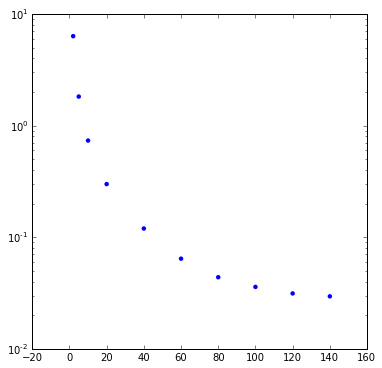In :
```mds3 = manifold.MDS(n_components=2, random_state=3, dissimilarity="precomputed",n_jobs = 4, verbose=0,max_iter=1000)
results3 = mds3.fit(dist_mat)
coords3=results3.embedding_
d = distCompare(dist_mat,coords3,nPicks=-1)
scatter([x for x,y in d],[y for x,y in d],edgecolors='none')
```
Out:
```<matplotlib.collections.PathCollection at 0xd9fc650>
```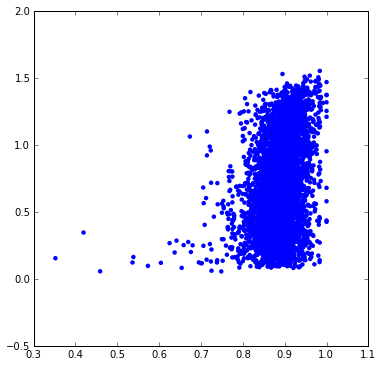Yeah, that's really bad.

# What about George's results?

In :
```TRGT = 'CHEMBL5024'
re = requests.get('https://www.ebi.ac.uk/chemblws/targets/{0}/bioactivities.json'.format(TRGT))
data = pd.DataFrame(re.json()['bioactivities'])
data = data.drop_duplicates(['parent_cmpd_chemblid'])
assays = data[['assay_chemblid','target_chemblid']].groupby('assay_chemblid').count()
goodassays = list(assays.ix[assays.assay_chemblid >= 4].index)
data = data.ix[data.assay_chemblid.isin(goodassays)]
data.shape
```
Out:
```(124, 16)
```
In :
```def fetch_SMILES2(chemblid):
return str(requests.get('https://www.ebi.ac.uk/chemblws/compounds/{}.json'.format(chemblid)).json()['compound']['smiles'])
data['SMILES'] = data['parent_cmpd_chemblid'].map(fetch_SMILES2)
PandasTools.AddMoleculeColumnToFrame(data, smilesCol = 'SMILES')
```
In :
```mols = data[['parent_cmpd_chemblid','name_in_reference','SMILES', 'ROMol', 'assay_chemblid']]
fps = [Chem.GetMorganFingerprintAsBitVect(m,2,nBits=2048) for m in mols['ROMol']]
dist_mat = []
for i,fp in enumerate(fps):
dist_mat.append(DataStructs.BulkTanimotoSimilarity(fps[i],fps,returnDistance=1))
dist_mat=numpy.array(dist_mat)
print dist_mat.shape
```
```(124, 124)

```
In :
```vs={}
for nComps in (140,120,100,80,60,40,20,10,5,2):
mds3 = manifold.MDS(n_components=nComps, random_state=3, dissimilarity="precomputed",n_jobs = 4, verbose=0,max_iter=1000)
results3 = mds3.fit(dist_mat)
print 'Final stress at dim=%d:'%nComps,results3.stress_,'stress per element:',results3.stress_/len(dist_mat)
vs[nComps]=results3.stress_/len(dist_mat)
scatter(vs.keys(),vs.values(),edgecolors='none')
yscale('log')
```
```Final stress at dim=140: 2.08121181026 stress per element: 0.0167839662118
Final stress at dim=120: 2.1037541866 stress per element: 0.0169657595693

```See if we can improve things by adjusting the MDS tolerance.

In :
```vs2={}
for nComps in (140,120,100,80,60,40,20,10,5,2):
mds3 = manifold.MDS(eps=1e-8,n_components=nComps, random_state=3, dissimilarity="precomputed",n_jobs = 4,verbose=0,max_iter=1000)
results3 = mds3.fit(dist_mat)
print 'Final stress at dim=%d:'%nComps,results3.stress_,'stress per element:',results3.stress_/len(dist_mat)
vs2[nComps]=results3.stress_/len(dist_mat)
scatter(vs2.keys(),vs2.values(),c='r',edgecolors='none')
scatter(vs.keys(),vs.values(),edgecolors='none')

yscale('log')
```
```Final stress at dim=140: 0.127173227782 stress per element: 0.00102559054663
Final stress at dim=120: 0.128792973298 stress per element: 0.00103865301047

```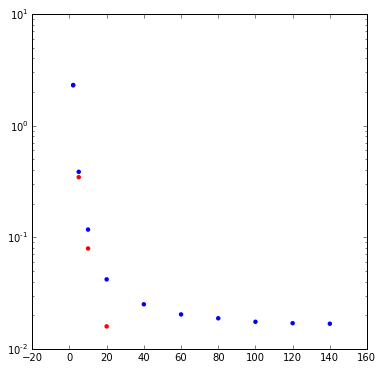That definitely helps at higher dimensions, but by the time we get down to 5D or 2D the improvement is mostly lost.

Try an even stricter eps value:

In :
```vs3={}
for nComps in (60,40,20,10,5,2):
mds3 = manifold.MDS(eps=1e-12,n_components=nComps, random_state=3, dissimilarity="precomputed",n_jobs = 4,verbose=0,max_iter=1000)
results3 = mds3.fit(dist_mat)
print 'Final stress at dim=%d:'%nComps,results3.stress_,'stress per element:',results3.stress_/len(dist_mat)
vs3[nComps]=results3.stress_/len(dist_mat)
scatter(vs.keys(),vs.values(),edgecolors='none')
scatter(vs2.keys(),vs2.values(),c='r',edgecolors='none')
scatter(vs3.keys(),vs3.values(),c='g',edgecolors='none')

yscale('log')
```
```Final stress at dim=60: 0.17771207137 stress per element: 0.00143316186589
Final stress at dim=40: 0.35550996703 stress per element: 0.00286701586315

```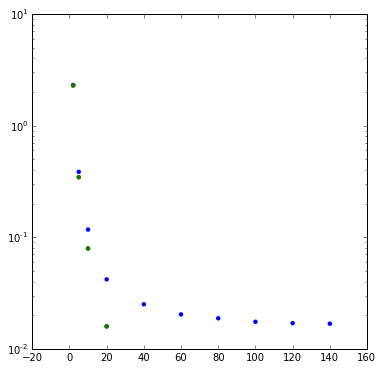No additional benefit there.

The fact that increasing the precision of the MDS doesn't help at all with embedding into 2 dimensions seems to be yet another sign that this data set really, really doesn't fit into 2D.

Embed into 2D and then look at the coords:

In :
```mds3 = manifold.MDS(eps=1e-8,n_components=2, random_state=3, dissimilarity="precomputed",n_jobs = 4, verbose=0,max_iter=1000)
results3 = mds3.fit(dist_mat)
coords3=results3.embedding_scatter([x for x,y in coords3],[y for x,y in coords3],edgecolors='none')
```
Out:
```<matplotlib.collections.PathCollection at 0xbfb8290>
```That's a similar picture to the one George found.

Here's the distance-distance plot:

In :
```d = distCompare(dist_mat,coords3,nPicks=-1)
scatter([x for x,y in d],[y for x,y in d],edgecolors='none')
```
Out:
```<matplotlib.collections.PathCollection at 0xbdd1950>
```That's not too terrible in at least one respect: things that are supposed to be reasonably close together (dists < 0.4) are close together.

Zoom in to the small-distance region and see what that looks like:

In :
```scatter([x for x,y in d],[y for x,y in d],edgecolors='none')
ylim((-.05,.5))
_=xlim((-.05,.5))
```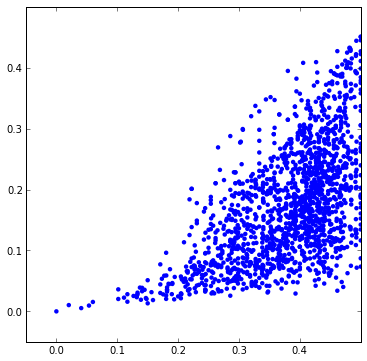Well, there's at least a correlation.

A summary of the situation: local environments in clusters (things close to each other) should be reasonably well represented, but the layout of the clusters relative to each other is probably not to be trusted.